Search IntMath
Close

450+ Math Lessons written by Math Professors and Teachers

5 Million+ Students Helped Each Year

1200+ Articles Written by Math Educators and Enthusiasts

Simplifying and Teaching Math for Over 23 Years

# Golden Spiral

By Murray Bourne, 04 Sep 2011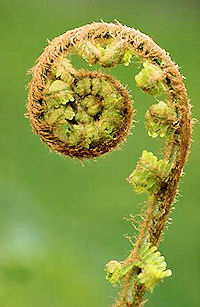Fern [image source]

Here's a recent question from reader Pehr in Sweden:

Hi,
First of all, wonderful site. Love it.
I've been studying the polar coordinates section hoping to expand my knowledge on the equiangular spiral. The interactive tools are great, though I'm having a hard time to derivate the exact mathematical solution to why the resulting function for the golden spiral is
r = ae^(b(theta))

For some background on Pehr's question, see:

Spirals are common in nature and have inspired mathematicians for centuries.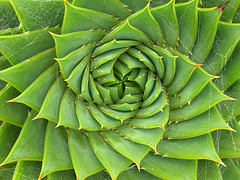Aloe spiral [Image source]Spiral galaxy NGC 5194
[Image courtesy NASA]

## Logarithmic Spirals

The Golden Spiral that Pehr is asking about is a special case of the logarithmic spiral.

Logarithmic spirals grow such that the angle of a line from the center of the spiral to the tangent to the curve at that point is constant. This is why they are also known as "equi-angular" spirals.

To see what this means, the 3 acute angles marked in the following fern image are approximately 80°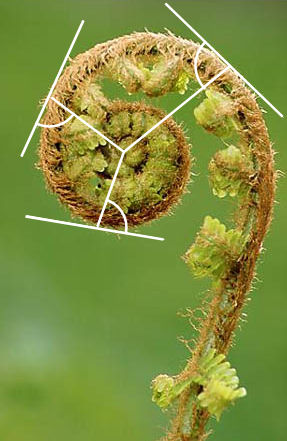Equi-angular fern

We normally use functions in Polar Coordinates when describing spirals. Otherwise, if we use ordinary rectangular coordinates, the formulas become very complex.

The formula for a logarithmic spiral using polar coordinates is:

r = aeθ cot b

where

r is the distance from the origin (or "pole")

a is a constant

θ is the angle (in radians) from the horizontal axis. So the coordinates of a point on the curve in polar coordinates is given by (r, θ).

b is the angle (in radians - the "equal" angle) that the line from the center of the spiral makes with the tangent to the spiral. In the fern case above, b ≈ 1.4 radians (≈ 80°).

As a consequence of the way we defined the logarithmic spiral, the ratio of the distances from the center to each spiral arm of an adjacent pair is constant.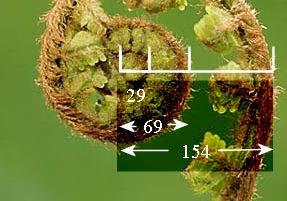Spiral arms at a constant ratio

The ratio

distance to the first arm: distance to second arm

= 29:69

≈ 0.42

The other ratio

distance to the second arm: distance to third arm

= 69:154

≈ 0.45

We see the ratios are almost the same. (In an actual logarithmic spiral, they are exactly the same. Choosing the start point for the fern is not an exact science!)

## Golden Spiral

The Golden Spiral is a special case of the logarithmic spiral.

We can write the general logarithmic spiral as a function in polar coordinates using t as follows:

r(t) = aet cot b

Note: Normally, we use θ for the independent variable, but we often use t as we can think of the spiral being traced out over time. Besides, it's easier to type!

The Golden Spiral has the special property such that for every 1/4 turn (90° or π/2 in radians), the distance from the center of the spiral increases by the golden ratio φ = 1.6180.

For this to occur, cot b must take the value (which comes from solving our function):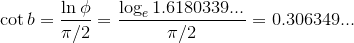Using this value, and taking the simple case where a = 1, our function becomes:

r(t) = e0.30635t

We'll use the excellent free graphing tool GeoGebra from here on.

## Setting up the Golden Spiral using GeoGebra

Now if we graph our function on ordinary rectangular coordinate axes in GeoGebra, we get the following exponential curve. Note that r increases at an ever-increasing rate (it gets steeper) as t increases.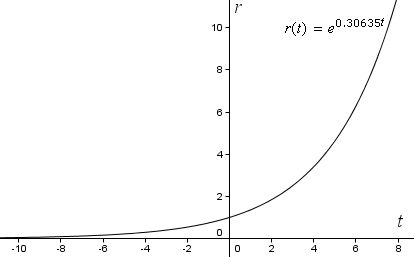But to see a spiral, we need to graph the curve using polar coordinates.

To convert the polar form (which we've got) to rectangular form (which we need for the graph) in Geogebra, we need to set up and graph the following function:

a(t) = (r(t) cos(t), r(t) sin(t))

Let's substitute a few important values to see what this expression means. Starting at t = 0, we get the starting point of the curve:

a(0) = (r(0) cos(0), r(0) sin(0))

= (1×1, 1×0)

= (1, 0)

So it means we start 1 unit from the origin along the positive x-axis. You can see the starting point in the following graph of the spiral.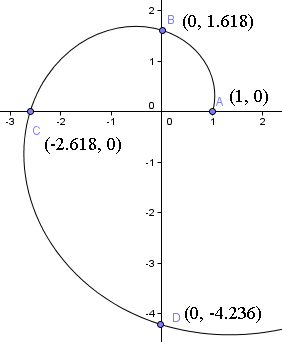Next, we rotate a quarter turn and find at t = π/2,

a(π/2) = (r(π/2) cos(π/2), r(π/2) sin(π/2))

= (1.618×0, 1.618×1)

= (0, 1.618)

Note that we are now 1.618 units from the origin up the y-axis. That is, φ = 1.6180 times the distance we started from.

Another rotation of a quarter turn brings us to t = π, where:

a(π) = (r(π) cos(π), r(π) sin(π))

= (-2.618×1, -2.618×0)

= (-2.618, 0)

We are now 2.618 units away from the origin along the negative x-axis, or φ = 1.6180 times the distance from the origin we were at the last quarter turn.

Note:

φ2 = 2.6180

We could work out our next position, along the negative y-axis, by just multiplying this last value by φ = 1.6180, giving us:

φ3 = 4.23606...

So the spiral will cut the y-axis at (0, -4.236).

One more quarter turn will bring us to φ4 = 6.85410... units along the positive y-axis, that is (6.854, 0).

We can see these values are correct on our spiral graph above.

If we keep going, we'll get a spiral as follows (this is 2 complete revolutions, or 4π = 720°):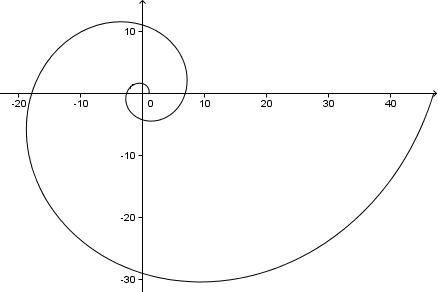As an aside, since in this problem

cot b = 0.30635

then

b = arccot 0.30635 = 1.274 radians or around 73°

This is the angle our spiral arms make with a line from the center of the spiral. You can see on the graph above each spiral arm makes an angle of 73° with the x-axis (and y-axis, or any line from the center).

## Approximating the Golden Spiral using arcs of a circle

We can obtain a spiral that looks quite similar to the Golden Spiral by using arcs of circles that increase in size by the Golden Ratio, as follows.

We start with a 1×1 square and draw an arc, center C, through 2 corners such that the sides of the square are tangent to the arc (that is, they touch once only).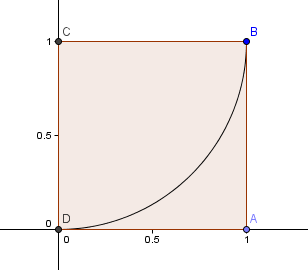Next, we place a square with side length φ = 1.6180 above our first square and construct another circular arc, center E, as before: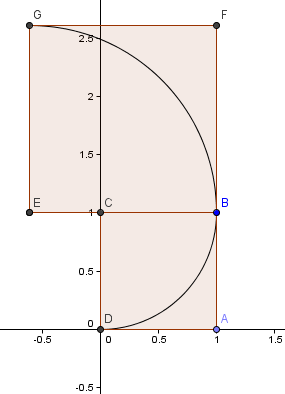Our next square goes on the left and has sides φ2 = 2.6180 = 1 + φ.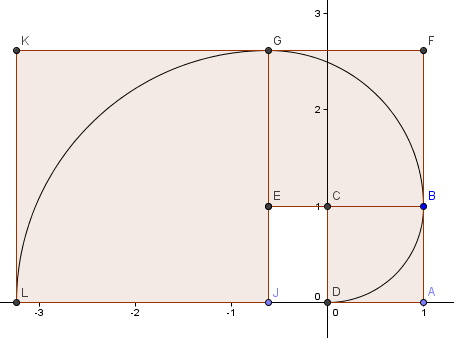We continue the pattern (we've gone another complete round) and get a spiral which looks quite a lot like our Golden Spiral from before.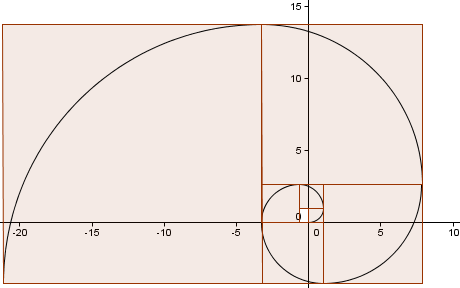## How close is our approximation?

Wikipedia's article on Golden Spiral has an image which claims the above spiral and the Golden Spiral are very close in shape.

Here's that image: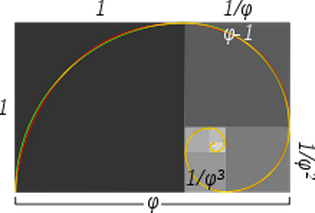The caption for the image states:

Approximate and true golden spirals: the green spiral is made from quarter-circles tangent to the interior of each square, while the red spiral is a golden spiral, a special type of logarithmic spiral. Overlapping portions appear yellow. The length of the side of a larger square to the next smaller square is in the golden ratio.

Can we re-create this ?

In the image below, the red curve is first part of the Golden Spiral we constructed above, whereas the green curve is based on the quarter-turn approximation we were just working on.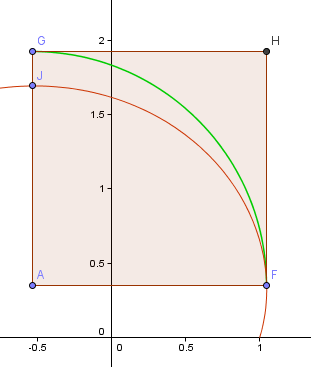The point F is the "right most" point on the spiral, which will be my starting point for the quarter-turn arc. The point J is the highest point of this portion of the spiral.

Point A is the intersection of the horizontal and vertical lines passing through F and J respectively and this will be the center of my arc.

Now, the arc GF is not at all close to the related portion of the spiral FJ.

Let's do another step and see if the next part is any better.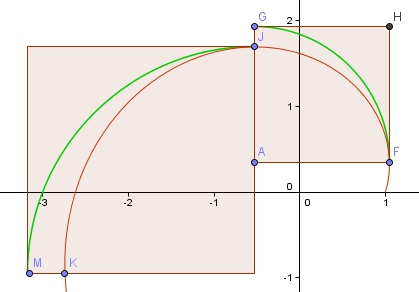As you can see, it's worse (as expected, since we have moved further away from the origin and the spiral arm is getting bigger).

Clearly, this is never going to work.

However, in my earlier Golden Spiral I was using:

r(t) = e0.30635t

The constant a, had value 1.

If we want our approximating arcs to be a good fit for the actual Golden Spiral, we need to use a value of (probably not surprisingly)

a = φ = 1.618103399..

This gives us the following curves, similar to the graph in Wikipedia.

The red curve is the Golden Spiral,

r(t) = 1.618013 e0.30635t

The green curve is the collection of circular arcs.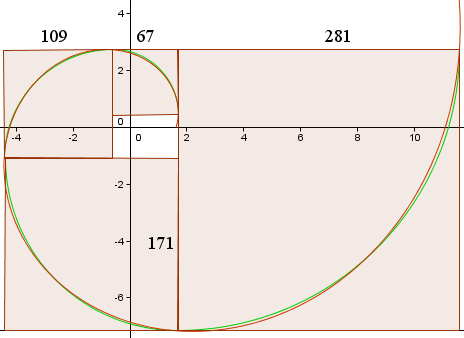The side length of the squares (in pixels) are shown and we can see they are approximately in the ratio 1.618013...

## Golden Spiral in the media

From Wolfram's Mathworld:

In the Season 4 episode "Masterpiece" (2008) of the CBS-TV crime drama "Criminal Minds," the agents of the FBI Behavioral Analysis Unit are confronted by a serial killer who uses the Fibonacci number sequence to determine the number of victims for each of his killing episodes. In this episode, character Dr. Reid also notices that locations of the killings lie on the graph of a golden spiral, and going to the center of the spiral allows Reid to determine the location of the killer's base of operations.

Here's more interesting information from Wolfram's Mathworld:

Logarithmic Spiral

## For the geeks - design using Golden Spiral

It is believed by many that designs using the Golden Ratio and Golden Spiral are pleasing to the eye.

Here is a great article by a guy who has contructed a golden spiral without images. (Mostly for those interested in Web design)

As he suggests in the article, an elephant's trunk is also close to the Golden Spiral.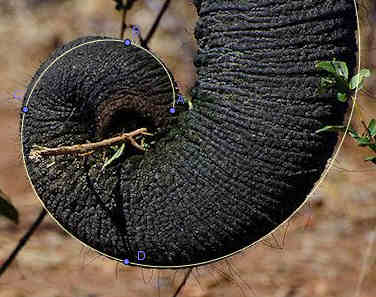Elephant trunk - almost a Golden Spiral

## Conclusion

The Golden Spiral is an interesting topic - one worth pursuing not only for the pleasant designs involved, but also the interesting math behind them.

### 21 Comments on “Golden Spiral”

1. Fibonacci joke « Random Walks says:

[...] Golden Spiral (squareCircleZ) [...]

2. Dave says:

How would one construct an accurate 3D golden spiral like you find in a pine cone?

3. Murray says:

Hi Dave. Interesting question! This PDF may give you some starting points: The Natural 3D Spiral.

If not, this search may help.

4. Mary says:

I want to draw a spiral such as the one in the elephant trunk in GeoGebra, but inside a pinecone. I can't find the way. Any ideas?

5. Murray says:

@Mary: GeoGebra allows you to place an image in the background, and then you could use the formula given in the post for your spiral. Is that what you are trying to do?

6. Maciek says:

I found the article that the red curve:

, which you claim is golden spiral doesn't fit perfectly the square area split (like on the picture).
See. http://www.emis.de/journals/NNJ/Sharp_v4n1-pt03.html

"If the spiral were to touch the sides of the rectangle, the line from the pole would need to make a tangent angle of 72.9676° with the side of the rectangle."

It says that an additional rotation (~3.75°) is needed to make it perfect.
Would you confirm that is the case?
and if so, would you know the math to calculate the exact value of that angle?

7. Murray says:

@Maciek: I haven't come across that issue before. Perhaps the author of that article could provide the missing steps for you?

8. Fibonacci Spirals - How to use them in daily trading - Trading Journals | futures io social day trading says:

[…] If you want to create your Fibo spiral, look here: Golden Spiral […]

9. kanil gunewardena says:

Like to have a symbolic solution for θ
in Equiangluar Spiral expression d = exp ^(θ . cot( αT ) . cos (θ )

to determine the spiral angle for a given d (x-coordinate) on spiral
This is for a 3D design purpose. αT is the Spiral TANGENT angle

Can I paste an image HERE to explain more ? How

Thanks

10. zac says:

@Kanil: I don't believe there is a "nice" solution for theta in this case. You would need to do it numerically. That is, create an array of (&theta, d) values by substituting θ, and then do reverse look up to find θ for a given d.

11. ??? says:

-- a(0) = (r(0) cos(0), r(0) sin(0))
--
-- = (1×1, 0×0)
--
-- = (1, 0)

I don't get this, how is r(0) = 1, then r(0) = 0?

What is r?

12. zac says:

Hello

Thanks for pointing out the typo which I have now corrected. The middle line should have been this:

= (1×1, 1×0)

It is now!

13. Jennifer Lee says:

Hello, two questions:
In the final diagram of the two overlapping spirals, Why are the side lengths for all the squares such huge numbers? For example, the smallest square looks like it should have a side length of around two but it is marked 67.
Second question: for the final diagram, the squares do not have the same growth factor, i.e. it isn't like in the first example where you obtained all the squares by multiplying the previous one's side lengths by phi. So since the squares are all different sizes, how did you know what size to make them?
Thank you.

14. Murray says:

@Jennifer: The side lengths of the squares in that overlapping spiral diagram are in pixels (since such units are easy to measure in image editing software). Sorry about the confusion - I've updated the post to say this is the case.

In the first one I was starting with a (unit) square then multiplying subsequently by φ, and constructing a spiral from circular arc based on those square. In that final one, I'm going the other way round - starting with a spiral based on an exponential function (the red one) and then constructing squares around that spiral, then measuring those squares to see if they do share the common ratio. (I also added the green "based on circular arcs" spiral for comparison.)

Hope it helps!

(BTW - Reader's comments only appear once I've approved them, to remove spam.)

15. Jennifer Lee says:

Thank you for your previous response. However, I'm still kind of confused about how you were able to construct the squares in the final graph though. If you didn't have a standard growth factor, then how did you calculate how big each square should be? Especially since the red spiral goes slightly outside some of the squares, so you couldn't have just plotted points on the widest parts of the spiral....

16. Murray says:

@Jennifer: Good on you for thinking this through carefully!

Let's consider the smallest square, marked 67px wide. What it appears I did (it's been 7 years since I wrote this piece, so I'm not exactly sure now) is to draw a vertical line through the right-most part of the spiral, and then a horizontal line through the top most part. The square was completed based on those extremities.

Then for the second largest square, the left side vertical line from the first square is extended to meet the horizontal drawn from the left-most extremity of the spiral. Completing that gives the 109px square. The red and green curves are close to each other - I probably chose the actual point where I'd get a square, so the green quarter-circle would fit each time.

17. Fibonacci Facts - Perk Epic says:

[…] Golden Spiral is a shape that makes sure of the balance of a picture. As a result, a picture achieves a more […]

18. The Painting Ant – Grave Innovations says:

19. Robert Ferraro says:

Murray, I read your post on the logarithmic spiral and it sounds like you know a lot about it. I have a theory that I need to prove is indeed possible with the application of a logarithmic spiral. It's potentially as ground breaking for science as General Relativity. I know that sounds almost laughable but when I share it with you you will see it is indeed plausible.

If I can prove the numbers work with a logarithmic or Golden spiral then the theory will work. I'm good at basic math but not proficient enough or knowledgeable enough to manipulate equations for logarithmic spirals. It might only take an hour of your time to crunch the numbers to see if it is indeed plausible.

Thanks.

20. Murray says:

@Robert: Feel free to post your theory on the IntMath Forum. Someone may be able to advise you further.

21. A Rare Fibonacci Day - Interactive Mathematics says:

[…] see them in nature – in the spirals of pineapples, sunflowers, succulents, and pinecones. Look closely enough and […]

### Comment Preview

HTML: You can use simple tags like <b>, <a href="...">, etc.

To enter math, you can can either:

1. Use simple calculator-like input in the following format (surround your math in backticks, or qq on tablet or phone):
a^2 = sqrt(b^2 + c^2)
(See more on ASCIIMath syntax); or
2. Use simple LaTeX in the following format. Surround your math with $$ and $$.
$$\int g dx = \sqrt{\frac{a}{b}}$$
(This is standard simple LaTeX.)

NOTE: You can mix both types of math entry in your comment.